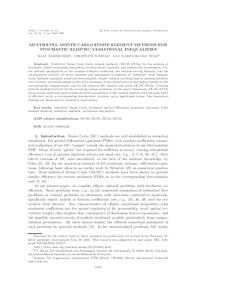Repository: Freie Universität Berlin, Math Department

# Multilevel Monte Carlo finite element methods for stochastic elliptic variational inequalities

Kornhuber, R. and Schwab, C. and Wolf, M.W. (2014) Multilevel Monte Carlo finite element methods for stochastic elliptic variational inequalities. SIAM Journal on Numerical Analysis, 52 (3). pp. 1243-1268. ISSN 0036-1429Preview

783kB

Official URL: http://dx.doi.org/10.1137/130916126

## Abstract

Multilevel Monte Carlo finite element methods (MLMC-FEMs) for the solution of stochastic elliptic variational inequalities are introduced, analyzed, and numerically investigated. Under suitable assumptions on the random diffusion coefficient, the random forcing function, and the deterministic obstacle, we prove existence and uniqueness of solutions of “pathwise” weak formulations. Suitable regularity results for deterministic, elliptic obstacle problems lead to uniform pathwise error bounds, providing optimal-order error estimates of the statistical error and upper bounds for the corresponding computational cost for the classical MC method and novel MLMC-FEMs. Utilizing suitable multigrid solvers for the occurring sample problems, in two space dimensions MLMC-FEMs then provide numerical approximations of the expectation of the random solution with the same order of efficiency as for a corresponding deterministic problem, up to logarithmic terms. Our theoretical findings are illustrated by numerical experiments.

Item Type: Article multilevel Monte Carlo, stochastic partial differential equations, stochastic finite element methods, multilevel methods, variational inequalities Mathematical and Computer Sciences > Mathematics > Numerical Analysis Department of Mathematics and Computer Science > Institute of Mathematics 1788 Ekaterina Engel 17 Feb 2016 09:32 03 Mar 2017 14:41

Repository Staff Only: item control page